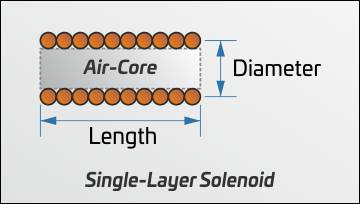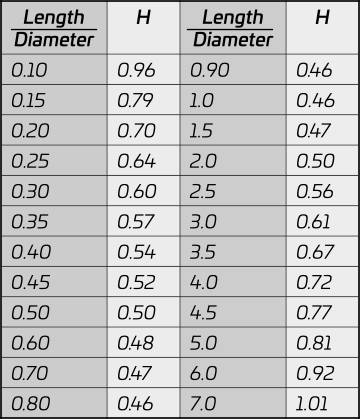# Coil Capacitance Calculator## Use the Coil Capacitance Calculator to calculate the self-capacitance of air-cored single-layer solenoid coils. With automated referencing and data interpolation for higher accuracy results

The formula for calculating the capacitance of an air-core single-layer solenoid is:$C= HD\; pF$

Where
D = Coil diameter in centimetres
H = Factor corresponding to coil length/diameter ratio in Table 1

Example: Calculate the self-capacitance of a coil that has a length of 15cm and diameter of 5cm$\frac{Length}{Diameter}=\frac{15}{5}=3$

Look up the number corresponding to a length to diameter ratio of 3 in Table 1:$H=0.61$

Substituting it into the equation:$C= 0.61*5=3.05\; pF$Coil dimensions for calculating capacitanceTable 1

### Using the Coil Capacitance Calculator

The Coil Capacitance Calculator automates the whole process and uses data interpolation to give you more accurate results.

Diameter: Enter the coil diameter, measured as depicted in the diagram above.

Length: Enter the coil length, measured as depicted in the diagram above.

Select Units: Select the units that you used for your coil dimensions from the list, and select your preferred output capacitance units.